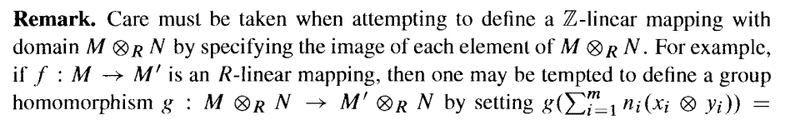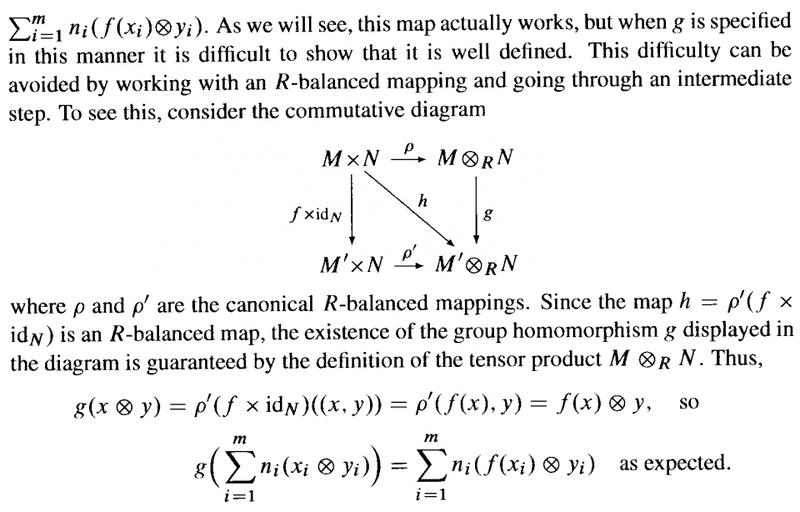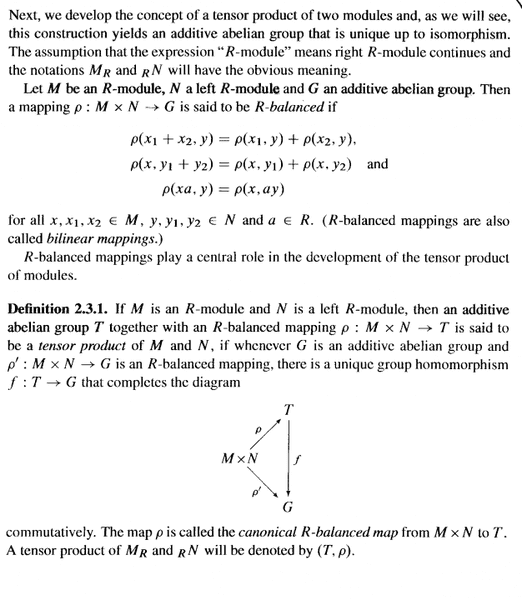# Tensor Products of Modules - Bland - Remark, Page 65

Gold Member
I am reading Paul E. Bland's book "Rings and Their Modules ...

Currently I am focused on Section 2.3 Tensor Products of Modules ... ...

I need some help in order to fully understand the Remark that Bland makes on Pages 65- 66Question 1

In the above text by Bland we read the following:

"... ... but when ##g## is specified in this manner it is difficult to show that it is well defined ... ... "

What does Bland mean by showing ##g## is well defined and why would this be difficult ... ...

Question 2

In the above text by Bland we read the following:

"... ... Since the map ##h = \rho' ( f \times id_N )## is an R-balanced map ... ... "

Why is ##]h = \rho' ( f \times id_N )## an R-balanced map ... can someone please demonstrate that this is the case?

Hope someone can help ... ...

Peter

=============================================================================

The following text including some relevant definitions may be useful to readers not familiar with Bland's textbook... note in particular the R-module in Bland's text means right R-module ...#### Attachments

Last edited:

andrewkirk
Homework Helper
Gold Member
Good morning MA. I wonder if you are affected by the wild weather this morning from the East Coast Low in the Tasman Sea.

In the example given, the purported definition of $g$ is that $g(x\otimes y)=f(x)\otimes y$. If we think about what this means, it is saying that

$$g\Big(\chi_{(x,y)}+K\Big)=\chi_{(f(x),y)}+K'$$

where $K$ and $K'$ are the submodules of $Z$ and $Z'$ respectively that have the required 'zero' properties inherited from the modules $M,M',N$; and $Z$ and $Z'$ are the free R-modules generated by ${\{\chi_{(u,v)}\ :\ u\in M\wedge v\in N\}}$ and ${\{\chi_{(u,v)}\ :\ u\in M'\wedge v\in N\}}$ respectively.

For this to be well-defined, we require that, for any $a,c\in M$ and $b,d\in N$ such that $(a,b)$ and $(c,d)$ are in the same coset of $K$, it will be the case that $(f(a),b))$ and $(f(c),d)$ are in the same coset of $K'$. Otherwise, $g$ will not be a function because $g\Big(\chi_{(a,b)}+K\Big)\neq g\Big(\chi_{(c,d)}+K\Big)$ even though $\chi_{(a,b)}+K=\chi_{(c,d)}+K$. Hence we have two different output values for a single input.

The argument involving the diagram appears to be a proof that $g$, thus defined, is indeed well-defined.

•Math Amateur
Gold Member
Hi Andrew ... thanks for helping ///

I am currently in regional Victoria ... back in Tasmania soon ... weather wet and cold here but not wild like around Sydney and the NSW coastline ...

Regarding you post ... yes, understand ... so what you are saying is ... ... that when Bland talks about ##g## being "well defined" he means that if we choose a different element ... say, ##\sum_{ i = 1}^m n'_i ( x'_i \otimes y'_i )## in the same coset as ##\sum_{ i = 1}^m n_i ( x_i \otimes y_i )## ... ... then ##g## still maps onto ##\sum_{ i = 1}^m n_i ( f(x_i) \otimes y_i ) ## ... ... is that correct ...?

andrewkirk
Homework Helper
Gold Member
Yes, that's right.

•Math Amateur
Gold Member
Yes, that's right.

Thanks Andrew ...

Peter